# Theorem Lesson Plan For B.Ed/DELED And School Teachers

0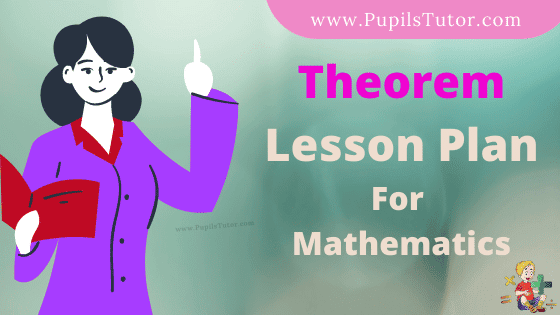Hello Friends, Welcome To Our Website. How Are You? Hope You Are Doing Well,

If You Are Searching For Theorem Lesson Plan Then You Have Come To The Right Place. Here We Have Shared The Lesson Plan On Theorems For B.Ed, D.El.Ed, And School Teachers.

This Real School Teaching Mathematics Lesson Plan On Theorem Is Specially Made For The B.Ed 1st And 2nd Year Students But All The School Teachers And Trainee Teacher Of All Classes Can Prepare Their Daily Teaching Plan Very Easily With The Help Of This Sample Math Theorem Lesson Plan.

Brief Overview Of The Lesson Plan

 Class 7th, 8 ,9 Th And 10 Topic Theorem Subject Maths Lesson Plan Type School Teaching Skill Real School Teaching

The Topics And Points That Are Covered In This Math Lesson Plan On Theorem Are : Different Mathematical Theorems, SAS Congruence Theorem Etc…..

## Theorem Lesson Plan –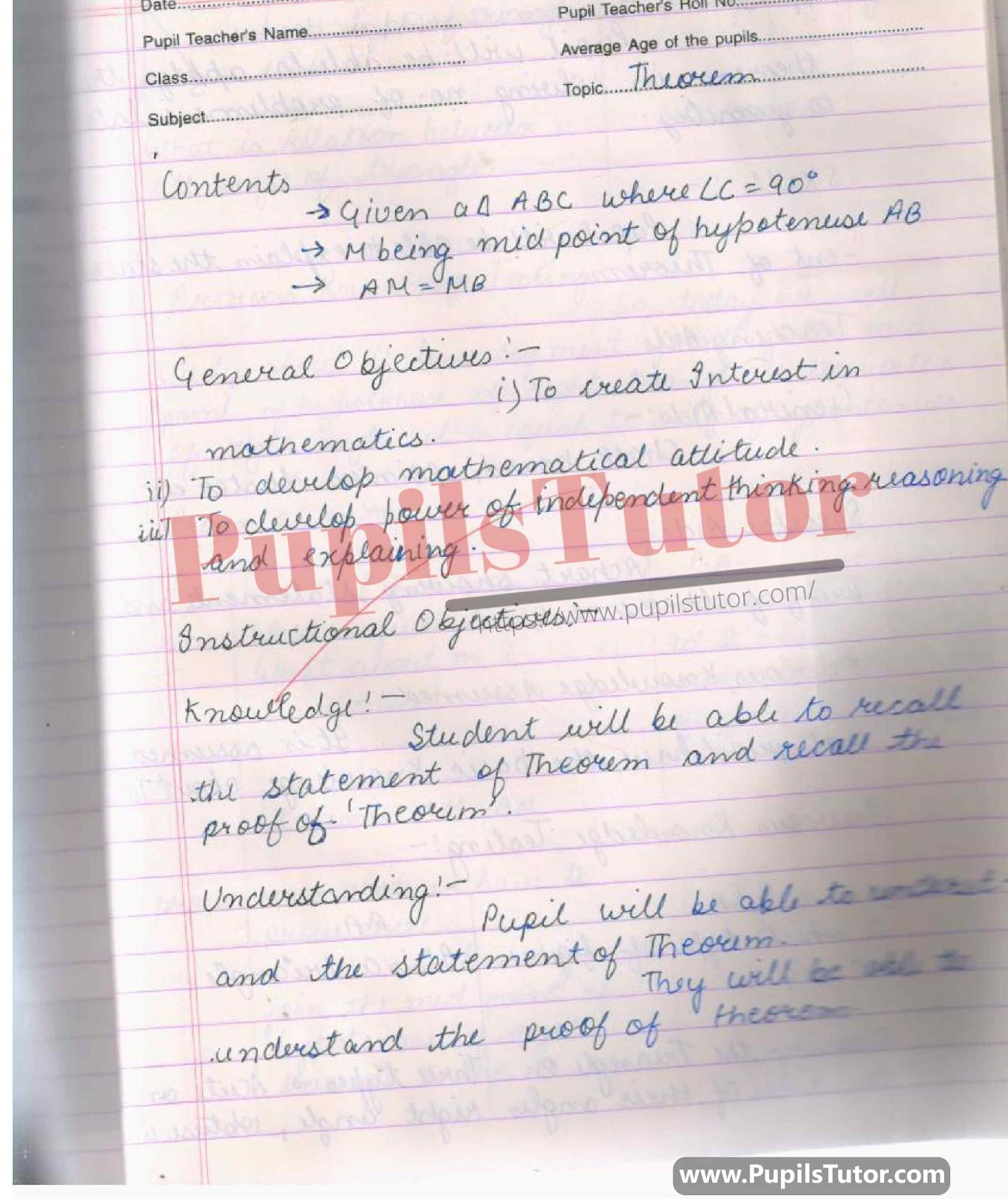## School Teaching Sample Lesson Plan Of Math On Theorems, Side Angle Side Congruency Theorem For B.Ed And Deled In English Free Download PDF And PPT (Power Point Presentation And Slides) –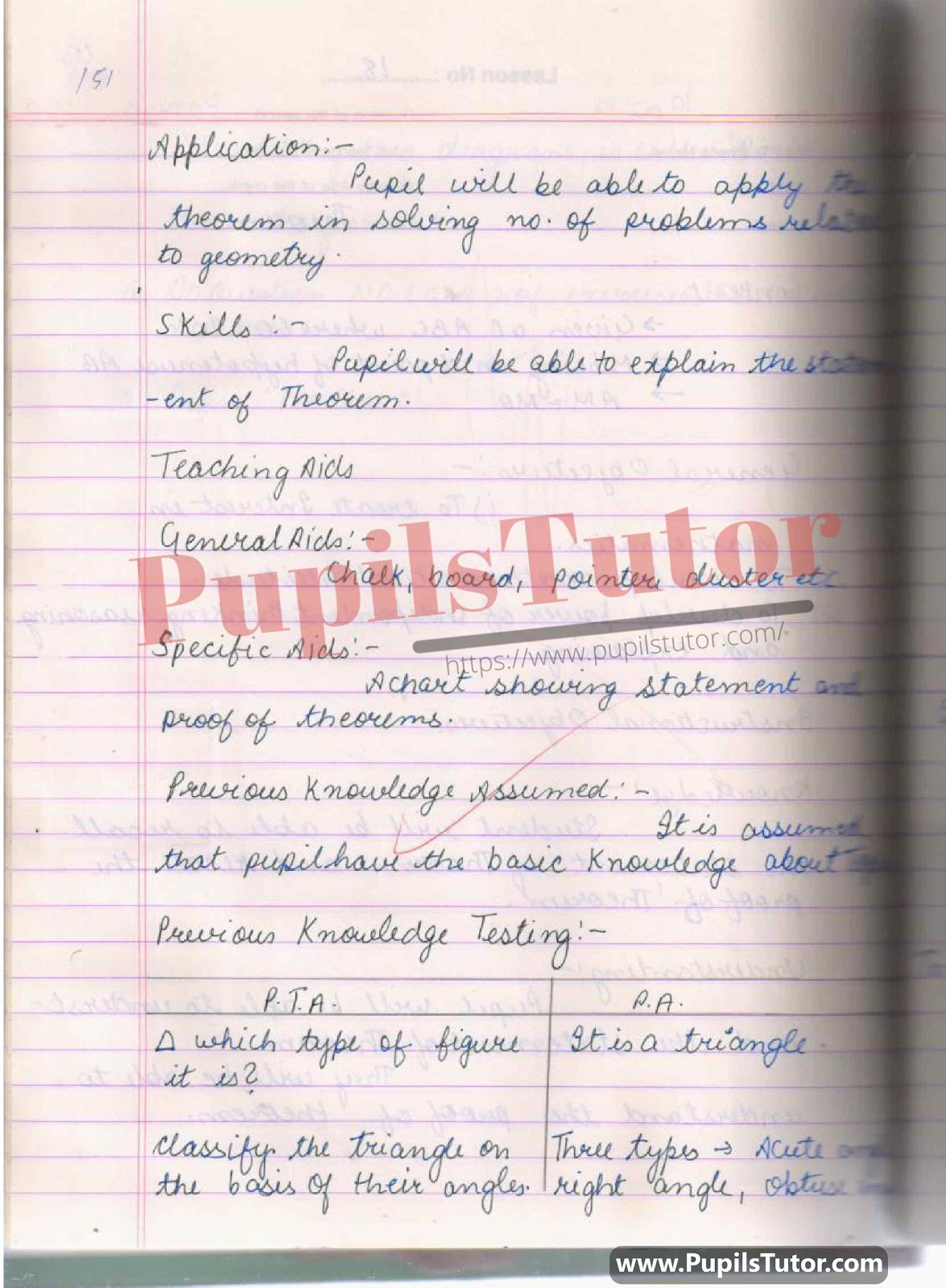## Math Lesson Plan On Theorem, Type Of Triangle, Hypotenuse, Perpendicular, Base For Class/Grade 7th, 8 ,9 Th And 10 For CBSE NCERT School And College Teachers –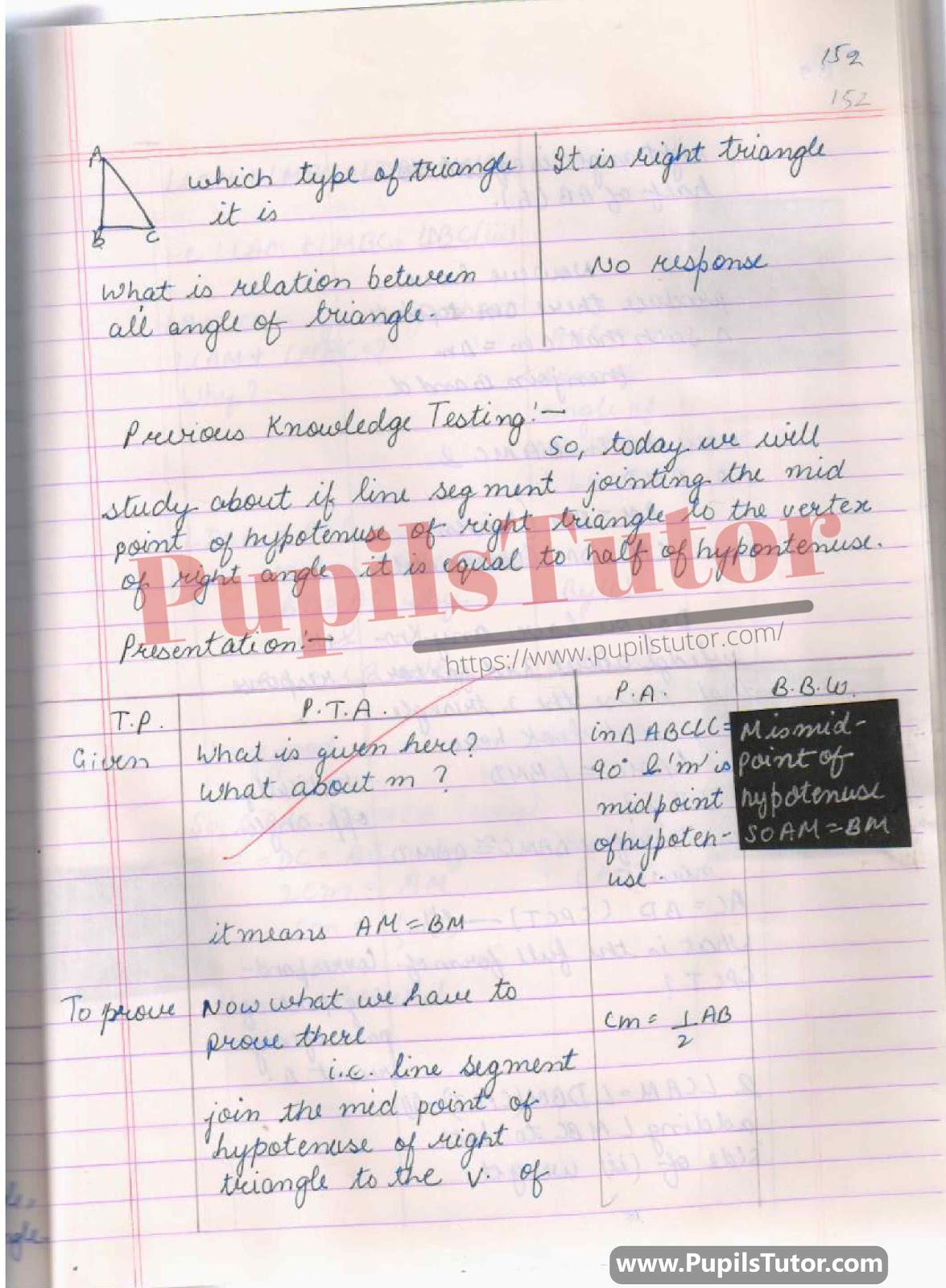## BED, DELED, BTC, BSTC, M.ED, DED And NIOS Teaching Of Maths Innovative Digital Lesson Plan Format On SAS Theorem, Congruency Of Triangle Topic For Class 9th, 10th, 11th, 12th –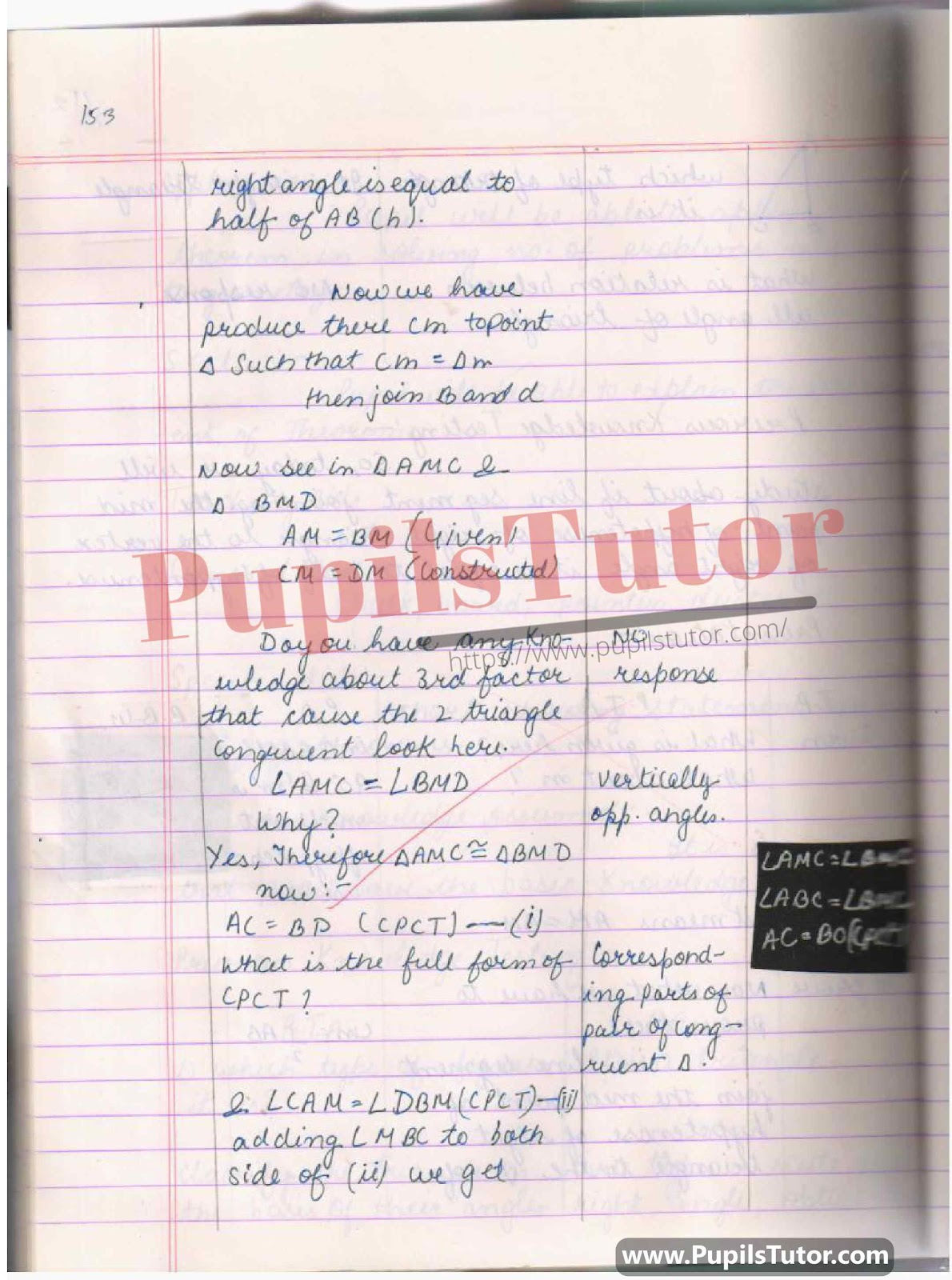## Math Lesson Plan On Different Theorem For Class 9 Th And 10th. –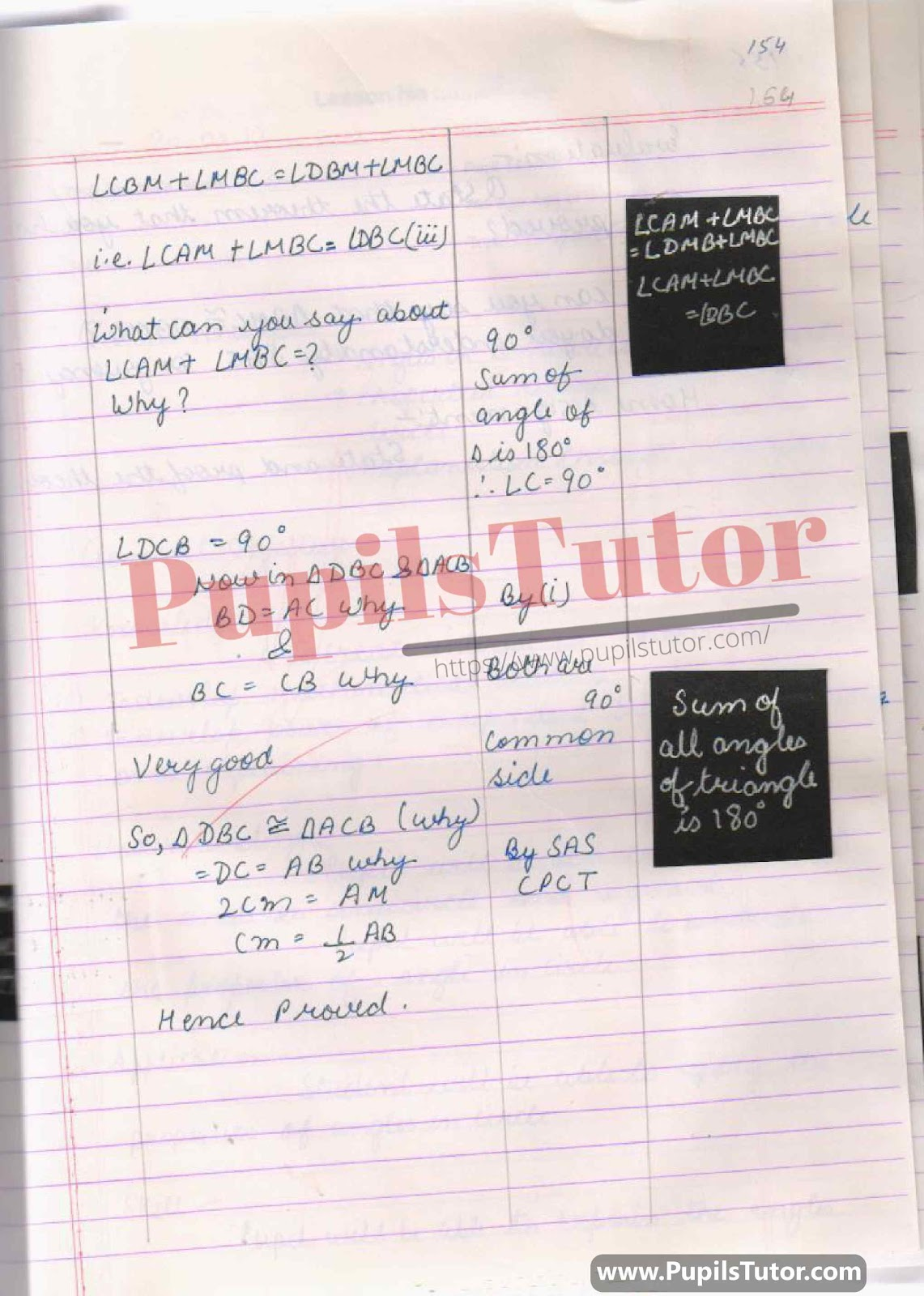## Theorem Lesson Plan For B.Ed 1st Year, 2nd Year And All Semesters Students –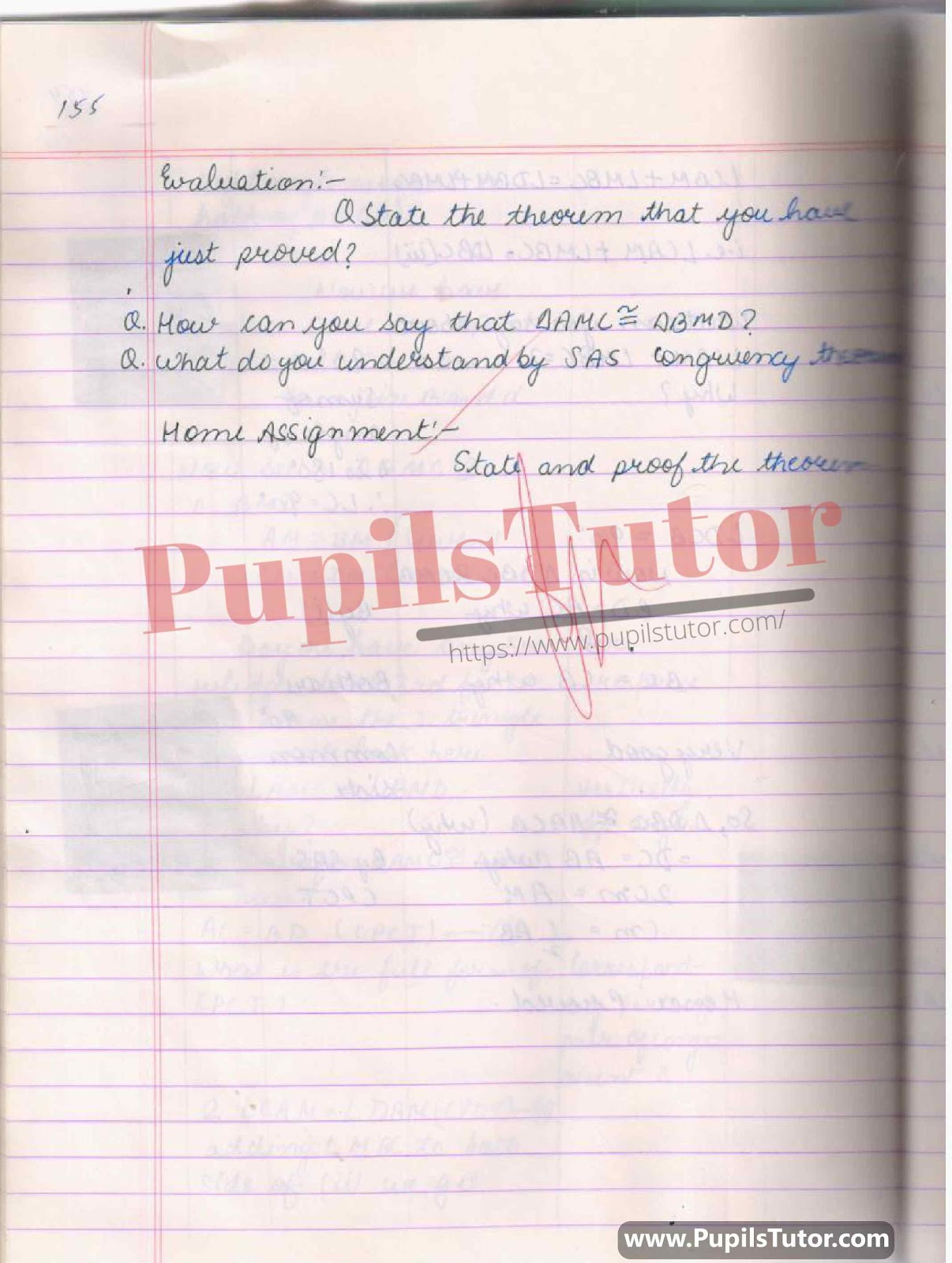You Might Also Like:

Further Reference

 B.Ed. Question Bank And Exam Notes A Complete Guide For B.ED. Entrance Examination CTET & TETs Preparation Material Teaching Aptitude and Attitude Test Book UP B.Ed Guidebook & Latest Practice Sets Book For Entrance Exam B.Ed CET Delhi University B.Ed Entrance Test Latest Practice Sets IGNOU B.Ed. Entrance Test: Previous Years Papers (Solved) Assignments And Files Lesson Planning Files And Guide

On Our Website www.PupilsTutor.Com, We Have Also Shared Lots Of Other Lesson Plans Of All Subjects On Various Teaching Skills Like Micro-Teaching, Mega Teaching, Discussion Skill, Real School Teaching And Practice Skill, Simulated And Observation Skills. You Can Also Check Them.

If You Liked This Theorem Lesson Plan For Class 7th, 8 ,9 Th And 10 Then Please Share Our Efforts With Your Friends Also.

You Can Also Share Your Lesson Plans, Assignments, Files, Papers And Study Notes With Us To Help Other Students .

#### List Of Some More Mathematics Lesson Plans

 Profit And Loss Math Lesson Plan For B.Ed And DELED Sum Of The Angles Of A Triangle Math Lesson Plan For B.Ed And DELED Trigonometric Ratio Math Lesson Plan For B.Ed And DED Circle Math Lesson Plan For B.Ed And DELED Properties Of Angle In Circle Math Lesson Plan For B.Ed Pie Chart Math Lesson Plan For B.Ed And DELED Three Dimensional Shape Math Lesson Plan For B.Ed And DELED Trigonometry Math Lesson Plan For B.Ed And DELED Parts Of A Circle Math Lesson Plan For B.Ed And DELED Compound Interest Math Lesson Plan For B.Ed And DELED Volume Of Cone And Cylinder Math Lesson Plan For B.Ed And DELED Areas Related To Circle Math Lesson Plan For B.Ed And DELED Integers Math Lesson Plan For B.Ed And DELED Surface Area Of Cone And Cylinder Math Lesson Plan For BEd And DELED Statistic & Arithmetic Mean Math Lesson Plan For B.Ed And DELED Volume Of Right Circular Cylinder Math Lesson Plan For B.Ed And DELED Circular Trigonometry Function Math Lesson Plan For B.Ed And DELED 3D Shapes Math Lesson Plan For B.Ed And DELED Coordinate Geometry Section Formula Maths Lesson Plan For B.Ed And DELED Surface Area & Volume Of Sphere Math Lesson Plan For B.Ed And DELED Theorem Math Lesson Plan For B.Ed And D.EL.ED Curved And Total Surface Area Of Cylinder Math Lesson Plan For B.Ed And DELED Quadrilateral Math Lesson Plan For B.Ed And DELED Height And Distance Math Lesson Plan For B.Ed And DELED Curved And Total Surface Area Of Cone Math Lesson Plan For B.Ed And DE.L.ED Coordinate Geometry Mathematics Lesson Plan For B.Ed And DELED Similar Triangle Math Lesson Plan For B.Ed And DELED Polynomials Math Lesson Plan For B.Ed And DELED Trigonometry Transformation Formula Math Lesson Plan For B.Ed And DELED Surface Area Of Cube And Cuboid Math Lesson Plan For B.Ed And DELED Algebraic Expression Math Lesson Plan For B.Ed And DELED Proportions Math Lesson Plan For B.Ed And DELED